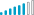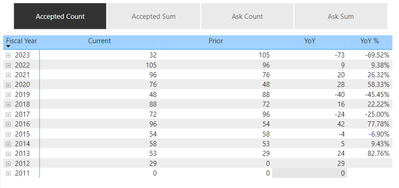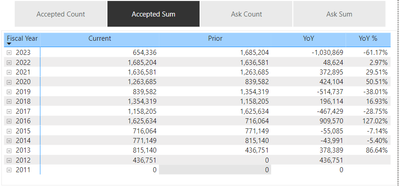cancel
Showing results for
Did you mean:Kudo Collector

## Tabular Editor Format String Expression help

Hi.

I'm new to Tabular Editor and calculation groups, and I'm wondering if I might get some advice.

I have a table containing a dimension that controls dynamic measure selection in my PBIX. It looks like this:

``````IF(ISCROSSFILTERED('Measure Dimensions'[Dimension]),
SWITCH(TRUE(),
VALUES('Measure Dimensions'[Dimension]) = "Accepted Sum",[Accepted Amount],
VALUES('Measure Dimensions'[Dimension]) = "Expected Sum",[Expected Ask Amount],
VALUES('Measure Dimensions'[Dimension]) = "Accepted Count",[Accepted Ask Count],
VALUES('Measure Dimensions'[Dimension]) = "Expected Count",[Expected Ask Count],
BLANK()
),BLANK()
)``````

I followed some online tutorials to build out a Time Intelligence calculation group in Tabular Editor (TE). One of my takeaways there was that the format set for the calculation items in TE overrides the format set on the measure in the PBIX; this is fine given my current use case.

Where I'm at now is setting the format for the SELECTEDMEASURE() based on the name of the selected measure from my measure dimension table. So, if it's a count, the format should be (I think) "0,0;-0,0", and if it's a sum, it should be "\$0.00;-\$0.00".

Can I accomplish this via the Format String Expression in the Expression Editor in TE? If so, do I have to explicitly add the same DAX to the Format Expression for each of my calculation items?

Thanks for any tips you can pass long!

1 ACCEPTED SOLUTIONKudo Collector

Well, the (a) solution appears to be to not use SELECTEDMEASURENAME() in the Format String Expression box but to use the SELECTEDVALUE() from my Measure Dimensions table. Like:

``````VAR DOLLARS =
SWITCH(
TRUE(),
SELECTEDVALUE('Measure Dimensions'[Dimension]) = "Accepted Sum",1,
0
)
VAR SumFormat = ( "\$#,#.00")
VAR CountFormat = ( "(#,0)")
RETURN
IF (DOLLARS,SumFormat,CountFormat)``````

Thanks again for your time looking at this, @v-rongtiep-msft ! I really appreciate it and am sorry to have wasted it!

4 REPLIES 4Kudo Collector

Well, the (a) solution appears to be to not use SELECTEDMEASURENAME() in the Format String Expression box but to use the SELECTEDVALUE() from my Measure Dimensions table. Like:

``````VAR DOLLARS =
SWITCH(
TRUE(),
SELECTEDVALUE('Measure Dimensions'[Dimension]) = "Accepted Sum",1,
0
)
VAR SumFormat = ( "\$#,#.00")
VAR CountFormat = ( "(#,0)")
RETURN
IF (DOLLARS,SumFormat,CountFormat)``````

Thanks again for your time looking at this, @v-rongtiep-msft ! I really appreciate it and am sorry to have wasted it!Kudo Collector

So, I'm zeroing in(?). Here's what I've entered in the Format String Expression field for my "Current" Time Intelligence calculation item:

``````VAR DOLLARS =
SWITCH(
TRUE(),
SELECTEDMEASURENAME() = "Accepted Sum",1,
0
)
VAR SumFormat = ( "\$#,0.00" )
VAR CountFormat = ( "#,0")
RETURN
IF (DOLLARS,SumFormat,CountFormat)``````

And here's what it now looks like in my matrix when I select one of my "sum" measures:So the comma is working, at least; dunno yet why the \$ isn't displaying....Community Support

My sample measure.

``Measure = IF(MAX('Table'[Column1])>20,FORMAT(MAX('Table'[Column1]),"\$#,0.00"),FORMAT(MAX('Table'[Column1]),"#,0"))``

You can change you measure.

``````VAR DOLLARS =
SWITCH(
TRUE(),
SELECTEDMEASURENAME() = "Accepted Sum",1,
0
)
VAR SumFormat = FORMAT(MAX('Table'[Column]),"\$#,0.00")
VAR CountFormat = FORMAT(MAX('Table'[Column1]),"#,0")
RETURN
IF (DOLLARS,SumFormat,CountFormat)``````If it does not help, please provide more details with your desired output and pbix file without privacy information (or some sample data) .

Best Regards
Community Support Team _ Polly

If this post helps, then please consider Accept it as the solution to help the other members find it more quickly.Kudo Collector

Thank you for the reply, @v-rongtiep-msft . I think I did a poor job of explaining my desired final result; I apologize. It's a matrix visual and accompanying measure slicer. The measure slicer displays the values in the unrelated Measure Dimensions table in my PBIX:

``````Measure Dimensions = {
("Accepted Sum",1),
("Expected Sum",3),
("Accepted Count",4),
("Expected Count",6)
}``````

Which contains, in turn, a measure called Measure Selection:

``````Measure Selection =
IF(ISCROSSFILTERED('Measure Dimensions'[Dimension]),
SWITCH(TRUE(),
VALUES('Measure Dimensions'[Dimension]) = "Accepted Sum",[Accepted Amount],
VALUES('Measure Dimensions'[Dimension]) = "Expected Sum",[Expected Ask Amount],
VALUES('Measure Dimensions'[Dimension]) = "Accepted Count",[Accepted Ask Count],
VALUES('Measure Dimensions'[Dimension]) = "Expected Count",[Expected Ask Count],
BLANK()
),BLANK()
)``````

Each of the measures referenced there is from my main Measures table in my PBIX. I created a Time Intelligence calculation group with a couple calcuation items that I'd like to display in my matrix. When I select a measure in my slicer that's a count, I want the format to be "#,0". When I select a measure that's a sum, I want the format to be "\$#,#.00".

So when I make this selection, I expect to see whole numbers with a comma (where applicable):When I make this selection, I'd like to see floats out to 2 digits and a dollar sign:FWIW, the YoY% format is set via the Format String Expression in the YoY% calculation item in Tabular Editor:I've tried setting the Format String Expression for the "Current" calculation item in Tabular Editor thusly:

``````VAR DOLLARS =
SWITCH(
TRUE(),
SELECTEDMEASURENAME() = "Accepted Sum",1,
0
)
//VAR SumFormat = ( "\$#,#.00") --excluded for testing
//VAR CountFormat = ( "(#,0)") --excluded for testing
RETURN
IF (DOLLARS,"\$#,#.00","#,0")``````

and this expression does seem to be applying the comma (I made sure the settings for the measure in the PBIX are set to not display a comma), but it's not applying the \$ sign.

I was looking at this yesterday afternoon and wonder if my resolution is that I need to create another calculation group in Tabular Editor to house my measures, then use those measures in my matrix. I've only read through that article 5 times now, so I'll need some time to read it five more times, which is the average number of times I need to read a SQLBI article before I understand it. 🤣

Thanks again for taking the time to look at this issue.Announcements#### Exclusive opportunity for Women!

Join us for a free, hands-on Microsoft workshop led by women trainers for women where you will learn how to build a Dashboard in a Day!#### Power Platform Conference-Power BI and Fabric Sessions

Join us Oct 1 - 6 in Las Vegas for the Microsoft Power Platform Conference.Top Solution Authors
Top Kudoed Authors
Users online (2,213)